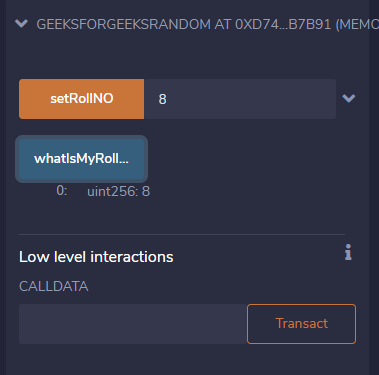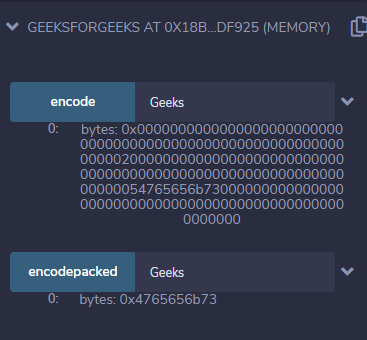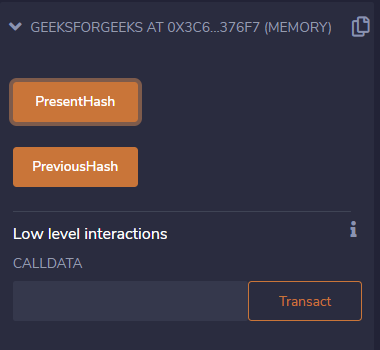Related Articles

# Solidity – Special Variables

There exist special variables and functions in solidity which exist in the global namespace and are mainly used to provide information about the blockchain or utility functions. They are of two types:

1) Block and Transaction Properties:

Note:

• The values of all members of msg can change for every external function call.
• block.timestamp, now and blockhash as a source of randomness are not secure. Timestamp and the blockhash can be influenced by the miners.

2) ABI encoding and decoding functions:

Example #1: In the below example, a contract is created to demonstrate msg.sender as a secure way to store the roll number.

## Solidity

 `// Solidity program to ` `// demonstrate msg.sender` `pragma solidity ^0.6.6;`   `// Creating a smart contract` `contract` `GeeksForGeeksRandom ` `{` `    ``// Creating a mapping` `    ``mapping (address => uint) rollNo;` `    `  `    ``// Defining a function to use ` `    ``// msg.sender to store roll no.` `    ``function setRollNO(uint _myNumber) ``public` `    ``{` `        ``// Update our 'rollNo' mapping ` `        ``// to store '_myNumber' under ` `        ``// 'msg.sender'` `        ``rollNo[msg.sender] = _myNumber;` `    ``}` `    `  `    ``// Defining a function to` `    ``// return the roll no.` `    ``function whatIsMyRollNumber()` `             ``public` `view returns (uint) ` `    ``{` `        ``// Retrieve the value stored ` `        ``// in the sender's address` `        ``// Will be `0` if the sender ` `        ``// hasn't called `setRollNO` yet` `        ``return` `rollNo[msg.sender];` `    ``}` `}`

Output:Example#2: In the below example, a contract is created with a function that demonstrates the variable abi.encode.

## Solidity

 `// Solidity program to ` `// demonstrate abi.encoding` `pragma solidity ^0.6.6;`   `// Creating a contract` `contract` `GeeksForGeeks ` `{    ` `    ``// Defining a function ` `    ``// to use abi.encode()` `    ``//It does padding to bytes` `    ``function encode(string memory g) ` `             ``public` `pure returns(bytes memory)` `    ``{` `        ``return` `abi.encode(g);` `    ``}` `    `  `    ``// encodepacked returns values in ` `    ``// a packed way without padding` `    ``function encodepacked(string memory g)` `             ``public` `pure returns(bytes memory)` `    ``{` `         ``return` `abi.encodePacked(g);` `    ``}` `}`

Input:

`Geeks`

Output:Example#3: In the below example, a contract is created to demonstrate the special variables block.number and blockhash.

## Solidity

 `// SPDX-License-Identifier: GPL-3.0 ` `pragma solidity >=0.5.0 <0.9.0;  ` `/// @title A contract for demonstrate block.number and blockhash` `/// @author Jitendra Kumar` `/// @notice For now, this contract just show how to return hash value of specific block` `contract` `GeeksForGeeks` `{` `    ``// Declaring state variables` `    ``// BlockNumber` `    ``uint BNumber;` `    `  `    ``// Hash of current block` `    ``bytes32 BHashPresent;` `    `  `    ``// Hash of Previous Block` `    ``bytes32 BHashPrevious;`   `    ``// Defining a function to` `    ``// return hash value of` `    ``// the current block` `    ``function PresentHash()` `            ``public` `returns(bytes32)` `    ``{` `        ``BNumber = block.number;` `        ``BHashPresent =blockhash(BNumber);` `        ``return` `BHashPresent;` `    ``}` `    `  `    ``// Defining a function to` `    ``// return the hash value of` `    ``// the previous block` `    ``function PreviousHash()` `            ``public` `returns(bytes32)` `    ``{` `        ``BNumber = block.number;` `        ``BHashPrevious =    blockhash(BNumber - 1);` `        ``return` `BHashPrevious;` `    ``}` `}`

Output:Whether you're preparing for your first job interview or aiming to upskill in this ever-evolving tech landscape, GeeksforGeeks Courses are your key to success. We provide top-quality content at affordable prices, all geared towards accelerating your growth in a time-bound manner. Join the millions we've already empowered, and we're here to do the same for you. Don't miss out - check it out now!